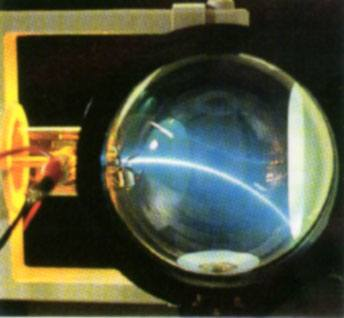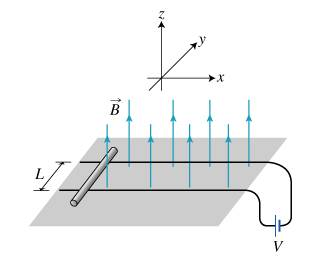# Change in electrons direction, changes the Lorentz force direction?

When the Lorentz force is applied on a wire that has current flowing in a magnetic field, by changing the direction of the electron(not it's KE) would this possibly change the direction of the Lorentz force? Can the wire be controlled in a way that the force's direction stays the same path?

Last edited:

mfb
Mentor
What do you mean with "initial"? In wires, net electron motions are always along the direction of the wire. You can rotate the wire to change the flow direction.
"stays the same path" as what?

jtbell
Mentor
Can the wire be controlled in a way that the force's direction stays the same path?

Apply a second force to the wire (e.g. from your hands!) so the net force on the wire is zero and it doesn't move.

What do you mean with "initial"?

edited.

In wires, net electron motions are always along the direction of the wire. You can rotate the wire to change the flow direction.
"stays the same path" as what?

Wouldn't the Lorentz force change the direction of the electron's velocity?
What would happen to the wire if the velocity direction is changed?

jtbell
Mentor
If you have a beam of electrons going through a vacuum without being inside a wire, a magnetic field causes the beam to curve (change direction):If the electrons are moving along a straight wire, then they can't follow that path, but are instead constrained to a straight path by the electrical attraction of the positive charges in the wire. And of course the electrons pull back on the positive charges (Newton's Third Law!). The net force on the wire (the magnetic force on the electrons) stays in one direction, perpendicular to the wire.

Last edited by a moderator:
Thank you for the help, that cleared A LOT of things...

Last edited by a moderator:
jtbell, what about comparing the Hall effect to the beam system presented above?
Don't the electrons move similar to that beam due to that conductor being fixed and not able to move?

Is it even possible that when a large conducting plate is placed in a magnetic field, and current flow where the plate starts to accelerate due to the Lorentz force... will the charges within the plate change direction and alter the Lorentz force path in any way?
What other forms of deceleration might that plate experience aside from the eddy currents and the mechanical resistances and the reduced current do to Faraday's law?

mfb
Mentor
You get a small, short-term net motion of charges, but this is completely negligible.

What other forms of deceleration might that plate experience aside from the eddy currents and the mechanical resistances and the reduced current do to Faraday's law?
Can you clarify which setup you have in mind here and where you expect a deceleration?

Like a rail gun concept:Or any other similar idea, where a conductor passes such a magnetic field.

I don't think its true that the Lorentz force direction will change as the conductor starts to accelerate?

mfb
Mentor
The force does not change its direction. The motion of the bar does not do anything: electrons and protons move with the same velocity. The only force you get is from the current flow, and that acts along the rails.

The force does not change its direction. The motion of the bar does not do anything: electrons and protons move with the same velocity. The only force you get is from the current flow, and that acts along the rails.

What about the motion of the bar induced motional emf = -vBL?

mfb
Mentor
I don't understand that question.

Jano L.
Gold Member
I don't think its true that the Lorentz force direction will change as the conductor starts to accelerate?

When the conductor changes velocity, the current density vector in the wire changes direction too and this makes the force
$$\int \mathbf j \times\mathbf B dV$$
change too. Besides the x component of that force which translates into accelerating force on the rod, there will be also y component of this force that counteracts the flow of current in the circuit.

When the conductor changes velocity, the current density vector in the wire changes direction too and this makes the force
$$\int \mathbf j \times\mathbf B dV$$
change too. Besides the x component of that force which translates into accelerating force on the rod, there will be also y component of this force that counteracts the flow of current in the circuit.

I don't understand what you mean, the conductor is a restrictor to the electrons they flow in a straight path as stated above, how can it's velocity change direction?

Jano L.
Gold Member
When the rod on the picture above stands still, the electrons move along the axis y. When the rod moves in the direction of axis x. The electrons move diagonally, in between the direction of y and x.

When the rod on the picture above stands still, the electrons move along the axis y. When the rod moves in the direction of axis x. The electrons move diagonally, in between the direction of y and x.

That will result in the change of the force's direction from the x axis?
What about the "constraints" that does not allow the electrons to do so? The constraint being the wire of course.

Jano L.
Gold Member
It is just vector addition of velocities. Wire moves along x. In the frame of the wire, the electrons move along y. Hence, in the lab frame the electrons move diagonally, partially along x, partially along y.

It is just vector addition of velocities. Wire moves along x. In the frame of the wire, the electrons move along y. Hence, in the lab frame the electrons move diagonally, partially along x, partially along y.

Not sure, what you point is honestly. As long as the wire is moving in the x direction due to the Lorentz force, that is the most important thing to me.
I doubt it changes direction, since the current will flow in the y axis, and can't "change" due to the conductor being it's constraint.

Is there anyway that the force acting on the wire would charge direction? Due to the bend of of charge in the wire caused by the Lorentz force?

A friend of mine argues that the change of charge's direction would change the force that would accelerate the conductor hence, reducing it in someway...
From the posts of other members(mfb, jtbell) it seems that notion is incorrect.